# #优质创作者# 深度学习基础-机器学习基本原理 原创 精华

1收藏

【本文正在参加优质创作者激励计划】

## 5.1 学习算法

### 5.1.1 任务 T

1. 分类：在这类任务中，计算机程序需要指定某些输入属于 $k$ 类中的哪一类，例如图像分类中的二分类问题，多分类、单标签问题、多分类多标签问题。
2. 回归：在这类任务中，计算机程序需要对给定输入预测数值。为了解决这个任务，学习算法需要输出函数 $f : \mathbb{R}^n \to \mathbb{R}$。除了返回结果的形式不一样外，这类 问题和分类问题是很像的。
3. 机器翻译
4. 结构化输出
5. 异常检测
6. 合成和采样
7. 去噪
8. 密度估计或概率质量函数估计
9. 输入缺失分类
10. 转录
11. 缺失值填补

### 5.1.3 经验 E

• 无监督(unsuper-vised)算法
• 监督(supervised)算法

### 5.1.4 示例: 线性回归

$$\hat{y} = w^{⊤}x \tag{5.3}$$

$$MSE_{test} = \frac{1}{m} \sum_{i}(\hat{y}^{(test)}-y^{(test)})_{i}^{2} \tag{5.4}$$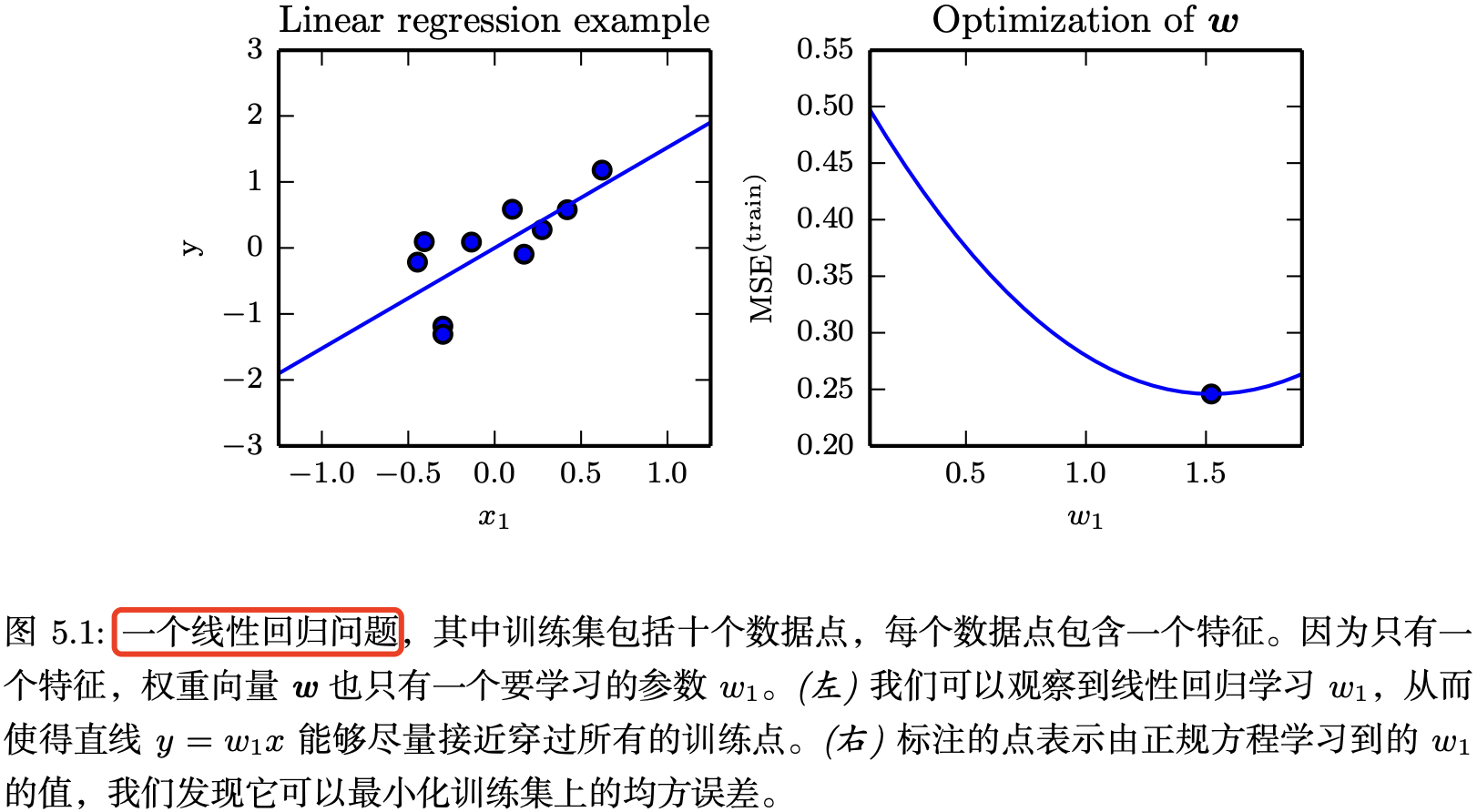## 5.2 容量、过拟合和欠拟合

• 欠拟合是指模型不能在训练集上获得足够低的误差。
• 而过拟合是指训练误差和和测试误差之间的差距太大。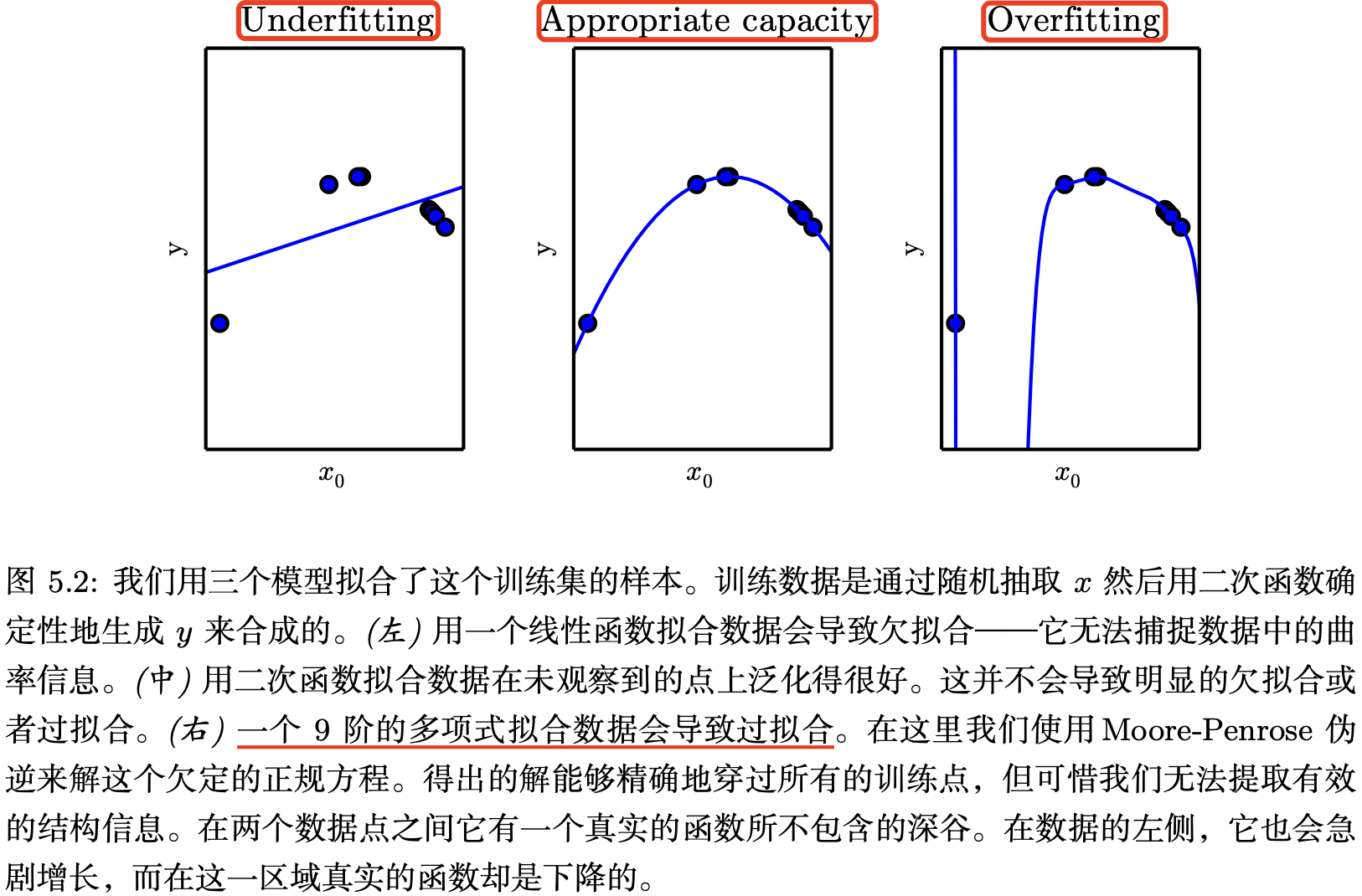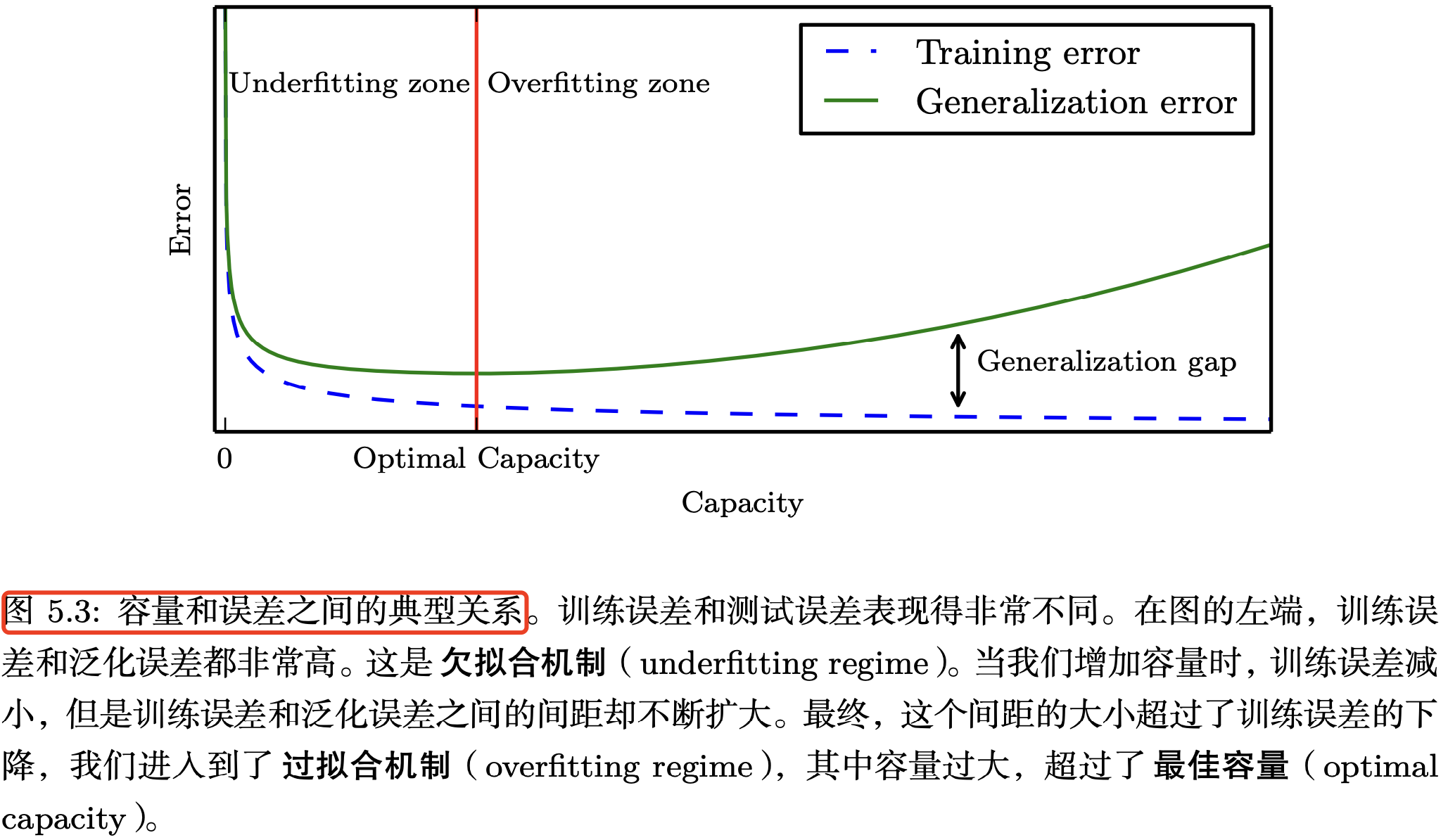### 5.2.2 正则化

• L1: $\lambda \times ||x||_{1}$，即所有元素的绝对值之和

• L2: $\lambda \times ||x||_{2}$，即所有元素的绝对值平方和

$$J(w,b)=\frac{1}{2m}\sum_{i=1}^m (z_i-y_i)^2 + \frac{\lambda}{2m}\sum_{j=1}^n{w_j^2}$$

$$J(w,b)= -\frac{1}{m} \sum_{i=1}^m [y_i \ln a_i + (1-y_i) \ln (1-a_i)]+ \frac{\lambda}{2m}\sum_{j=1}^n{w_j^2}$$

## 5.3 超参数和验证集

### 5.3.2 交叉验证

k 折交叉验证虽然一定程度上可以解决小数据集上测试误差的不确定性问题，但代价则是增加了计算量。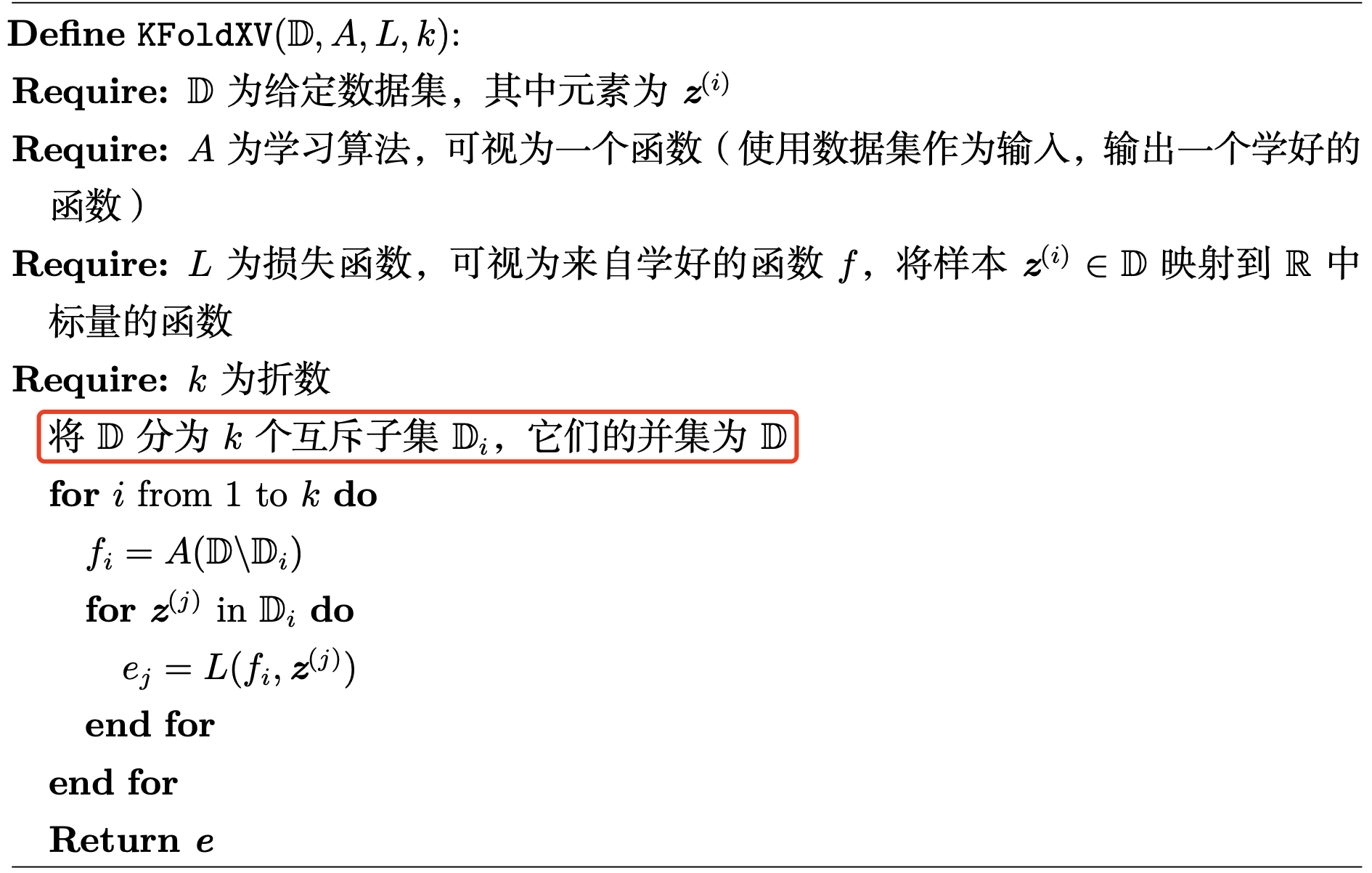## 5.4 估计、偏差和方差

### 5.4.1 点估计

$$\hat{\theta_m} =g(x^{(1)},…,x^{(m)}). \tag{5.19}$$

### 5.4.2 偏差

$$bias(\hat{\theta_m}) = E(\hat{\theta_m}) − \theta, \tag{5.19}$$

### 5.4.4 权衡偏差和方差以最小化均方误差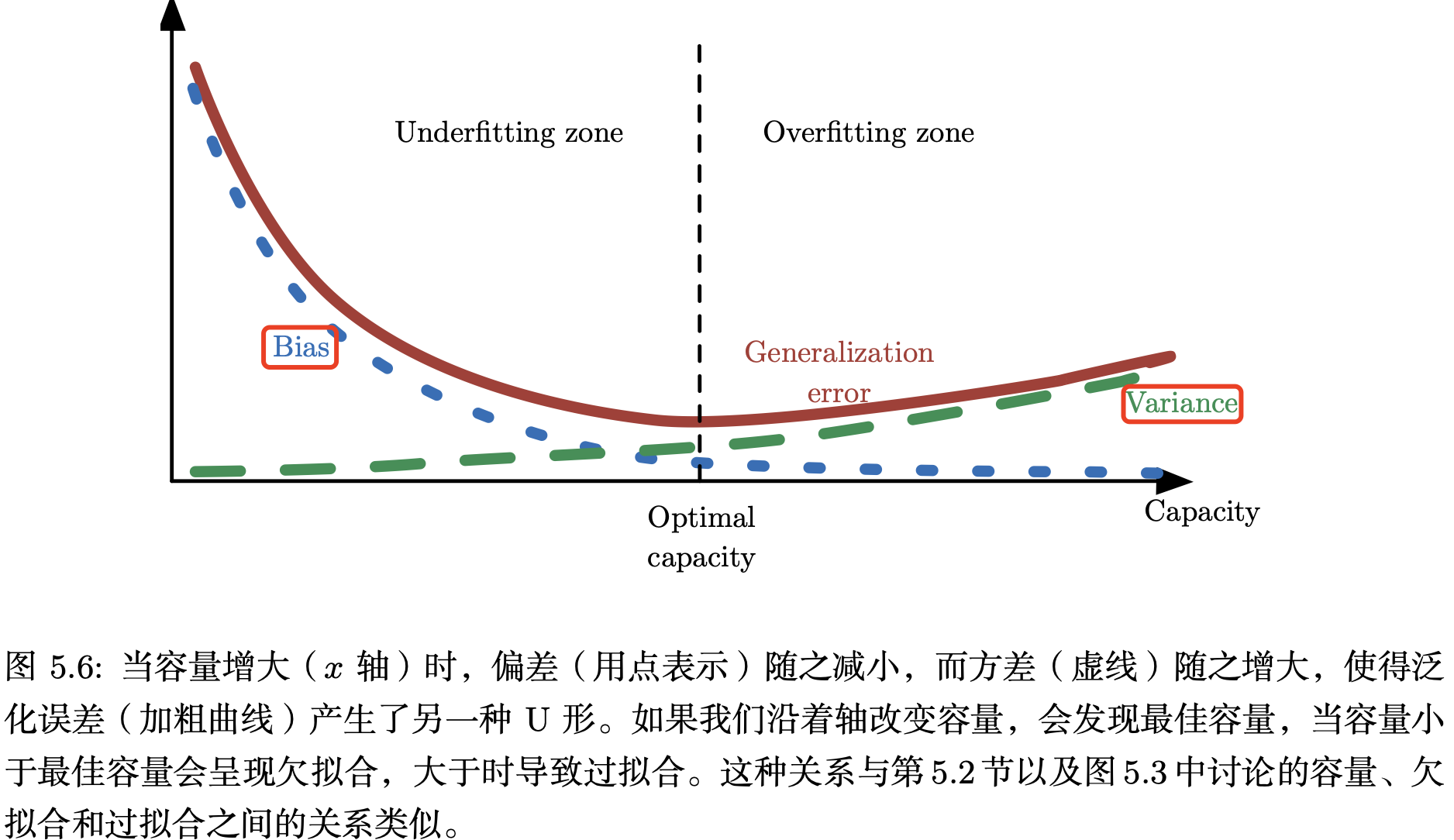## 5.8 无监督学习算法

### 5.8.1 PCA 降维

PCA（Principal Component Analysis）是学习数据表示的无监督学习算法，常用于高维数据的降维，可用于提取数据的主要特征分量。

PCA 的数学推导可以从最大可分型和最近重构性两方面进行，前者的优化条件为划分后方差最大，后者的优化条件为点到划分平面距离最小。

### 5.8.2 k-均值聚类

$k$-均值聚类初始化 k 个不同的中心点 ${μ^{(1)}, . . . , μ^{(k)}}$，然后迭代交换以下两个不同的步骤直到算法收敛。

1. 步骤一，每个训练样本分配到最近的中心点 $μ^{(i) }$ 所代表的聚类 $i$。
2. 步骤二，每一个中心点 $μ^{(i) }$ 更新为聚类 $i$ 中所有训练样本 $x^{(j)}$ 的均值。

## 5.9 随机梯度下降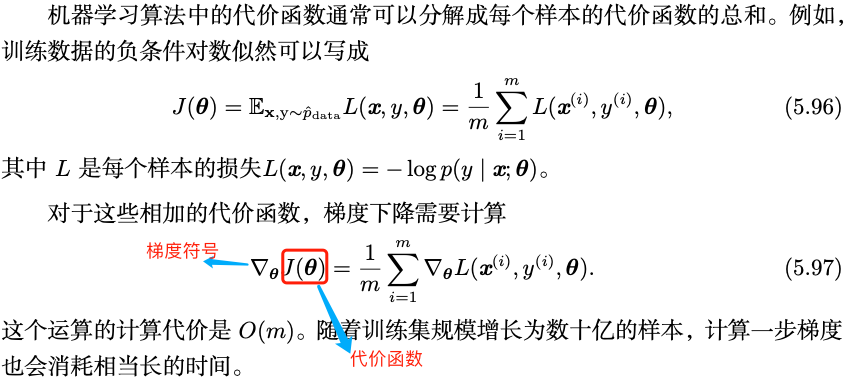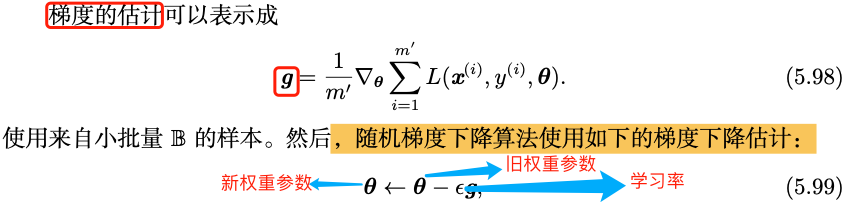1. 特定的数据集
2. 代价函数
3. 优化过程
4. 神经网络模型。

## 参考资料